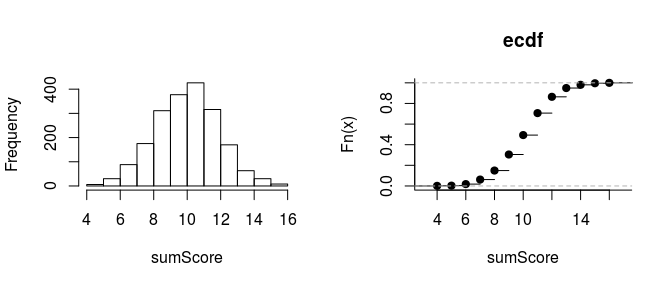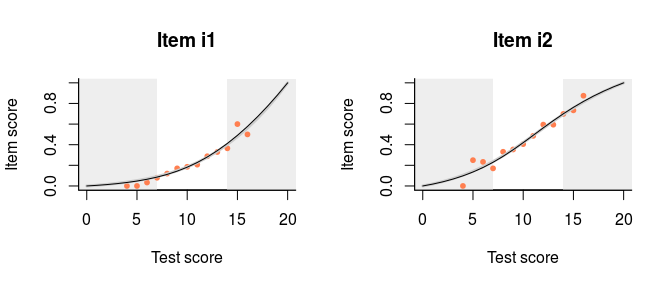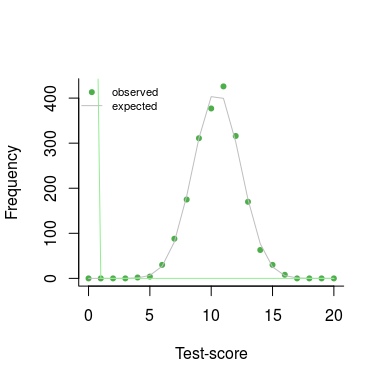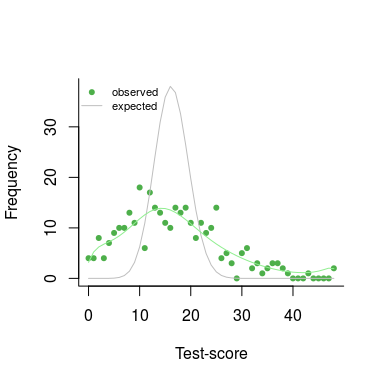# One theta to rule them all: Test Individual Differences

#### 16 december, 2017

Educational and psychological testing is all about individual differences. Using an instrument, we try our best to place an individual with respect to others depending on their level of extroversion, depression, or mastery of English.

What if there are no individual differences at all? We would find ourselves in the unenviable position of having no Snark to hunt for, no ghosts to bust. Dexter includes a function, individual_differences, to check whether the response data are consistent with the hypothesis of no individual differences in true ability.

Here is a simple function to simulate a matrix of response data from the Rasch model, in the long shape expected by dexter. We use it to generate responses to 20 items with uniformly distributed difficulties from 2000 persons having all the same true ability of 0.5:

sim_Rasch = function(theta, delta) {
n = length(theta)
m = length(delta)
data.frame(
person_id = rep(paste0('p',1:n), m),
item_id = rep(paste0('i',1:m), each=n),
item_score = as.integer(rlogis(n*m, outer(theta, delta, "-")) > 0)
)
}

simulated = sim_Rasch(rep(0.5, 2000), runif(20, -2, 2))

Computing the sum scores and examining their distribution, we find nothing conspicuous:

ss= simulated %>%
group_by(person_id) %>%
summarise(sumscore=sum(item_score))
par(mfrow=c(1,2))
hist(ss$sumscore, main='', xlab='sumScore') plot(ecdf(ss$sumscore), bty='l', main='ecdf', xlab='sumScore' )mm = fit_inter(simulated)

We can also examine the various item-total regressions produced by function fit_inter. For example, here are the plots for the first two items:

mm = fit_inter(simulated)
par(mfrow=c(1,1))
plot(mm, show.observed = TRUE,
items = c('i1','i2'),
nr=1, nc=2)The curtains that eliminate the 5% smallest and 5% largest sum scores are drawn somewhat narrow but, apart from that, all regressions look nice. It appears that, by just looking at the response data, we are not in a very good position to judge whether there are any true individual differences in ability. To help with that, dexter offers a function, individual_differences:

dd = individual_differences(simulated,degree=10)
## =
plot(dd)The gray line shows the predicted frequency of each sum score under the hypothesis of no true individual differences. The green dots show the observed frequencies and it will be clear that our observed data is compatible with the null hypothesis.

The print function for the test shows a chi-squared test for the null hypothesis. Note that this uses R’s option to simulate the p-value, which explains why the degrees of freedom are missing:

print(dd)
## Chi-Square Test for the hypothesis that all respondents have the same ability:
##
##  Chi-squared test for given probabilities with simulated p-value
##  (based on 2000 replicates)
##
## data:  observed
## X-squared = 12.303, df = NA, p-value = 0.6187

Thus, we find a p-value of 0.62 for the hypothesis that there are no individual differences.

What about real data? Dexter comes with a well-known example preinstalled, the verbal aggression data (Vansteelandt 2000) analysed in great detail in (Paul De Boeck 2004) and many others. 243 females and 73 males have assessed on a 3-point scale (‘yes’, ‘perhaps’, or ‘no’) how likely they are to become verbally aggressive in four different frustrating situations

db2 = start_new_project(verbAggrRules, "verbAggression.db")
add_booklet(db2, verbAggrData, "data")
## $items ##  "S1DoCurse" "S1DoScold" "S1DoShout" "S1WantCurse" "S1WantScold" ##  "S1WantShout" "S2DoCurse" "S2DoScold" "S2DoShout" "S2WantCurse" ##  "S2WantScold" "S2WantShout" "S3DoCurse" "S3DoScold" "S3DoShout" ##  "S3WantCurse" "S3WantScold" "S3WantShout" "S4DoCurse" "S4DoScold" ##  "S4DoShout" "S4WantCurse" "S4WantScold" "S4WantShout" ## ##$covariates
## character(0)
##
## $columns_ignored ##  "Gender" ## ##$auto_add_unknown_rules
##  TRUE
##
## <0 rows> (or 0-length row.names)
dd = individual_differences(db2, booklet_id=="data")
## ==
plot(dd)close_project(db2)